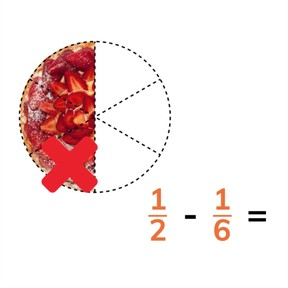Subtracting fractions with different denominators

# Subtracting fractions with different denominators

I can subtract fractions with different denominators.

No account needed.8,000 schools use Gynzy92,000 teachers use Gynzy1,600,000 students use Gynzy

## General

Students learn to subtract fractions with different denominators.

## Common core standard(s)

CCSS.Math.Content.5.NF.A

## Relevance

Discuss with students that it is useful to be able to subtract fractions with different denominators, so you can calculate how much you have left.

## Introduction

Show the fraction bars on the interactive whiteboard to the students and ask which fractions they are showing. If the fractions have a common denominator, students must subtract the fractions and find the difference. Repeat this with the cream pie example.

## Development

Explain to students how to subtract fractions with different denominators using visual support. 1//2 of a baguette is shown and 1//4 is taken away. These fractions have different denominators. You must first find a common denominator before you can subtract the fractions. To have a common denominator means that the denominators of both fractions has to be the same number. Show this with the given example. Next you can subtract the numerators to find the difference. Ask students to practice a few problems on their own with visual support. Tell students that when the denominator becomes twice as large, that the numerator also becomes twice as large. Tell students that solving subtraction problems with fractions with different denominators is exactly the same- you find a common denominator and then subtract numerators. In some cases, after you subtract, you can still simplify the difference. Have students practice subtracting fractions with different denominators without visual support. Finally discuss how to solve fraction word problems. They must first find the fractions, and then find a common denominator, and then can be solved. Ask students to practice the next fraction word problems individually or in pairs.

To check that students are able to subtract fractions with different denominators, ask them the following question:
- How do you make fractions with different denominators have a common denominator?

## Guided practice

Students are given subtraction problems with visual support, then without visual support, and are also given fraction word problems to solve. Remind students to simplify their differences.

## Closing

Remind students why it is important to be able to subtract fractions with different denominators, namely that you will then still be able to find the difference and determine how much is left. Show a few problems on the interactive whiteboard. Ask students to solve the problems and then find where the correct answer is on the interactive whiteboard. If all problems are answered correctly, the line should go from start to finish.

## Teaching tips

Students who have difficulty subtracting fractions with different denominators can start by practicing finding a common denominator for the fractions. Start with fractions in which the denominator of one fraction is double of the other. Students also need to remember to double the numerator. Then they can move on to practicing finding a common denominator for other fractions.

### The online teaching platform for interactive whiteboards and displays in schools

• Save time building lessons

• Manage the classroom more efficiently

• Increase student engagement